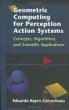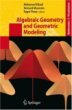# Maths - clifford / Geometric Algebra - Further ReadingClifford Algebra to Geometric Calculus: A Unified Language for Mathematics and Physics (Fundamental Theories of Physics). This book is intended for mathematicians and physicists rather than programmers, it is very theoretical. It covers the algebra and calculus of multivectors of any dimension and is not specific to 3D modelling.New Foundations for Classical Mechanics (Fundamental Theories of Physics). This is very good on the geometric interpretation of this algebra. It has lots of insights into the mechanics of solid bodies. I still cant work out if the position, velocity, etc. of solid bodies can be represented by a 3D multivector or if 4 or 5D multivectors are required to represent translation and rotation.Geometric Algebra for Physicists - This is intended for physicists so it soon gets onto relativity, spacetime, electrodynamcs, quantum theory, etc. However the introduction to Geometric Algebra and classical mechanics is useful.Geometric Computing for Perception Action Systems: Concepts, Algorithms, and Scientific Applications (Hardcover). This is the only book I have so far come across that has a reasonable explanation of 'motors' and why it is useful to use 4D Geometric algebra to represent kinematics of solid bodies (in chapter 2). The book is quite a slim volume considering that it covers both fundamental concepts and practical applications. Therefore I think you will need to have a good understanding of Geometric Algebra before starting on this book.Geometric Algebra for Computer Science: An Object-oriented Approach to Geometry. This book stresses the Geometry in Geometric Algebra, although it is still very mathematically orientated. Programmers using this book will need to have a lot of mathematical knowledge. Its good to have a Geometric Algebra book aimed at computer scientists rather than physicists. There is more information about this book here.Algebraic Geometry and Geometric Modeling: Proceedings of the Conference in Nice, September 04 (Mathematics & Visualization) (Hardcover).

Book Shop - Further reading.

Where I can, I have put links to Amazon for books that are relevant to the subject, click on the appropriate country flag to get more details of the book or to buy it from them.Clifford Algebra to Geometric Calculus: A Unified Language for Mathematics and Physics (Fundamental Theories of Physics). This book is intended for mathematicians and physicists rather than programmers, it is very theoretical. It covers the algebra and calculus of multivectors of any dimension and is not specific to 3D modelling.New Foundations for Classical Mechanics (Fundamental Theories of Physics). This is very good on the geometric interpretation of this algebra. It has lots of insights into the mechanics of solid bodies. I still cant work out if the position, velocity, etc. of solid bodies can be represented by a 3D multivector or if 4 or 5D multivectors are required to represent translation and rotation.

Other Math Books